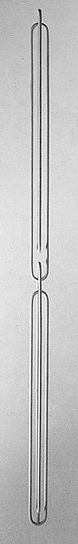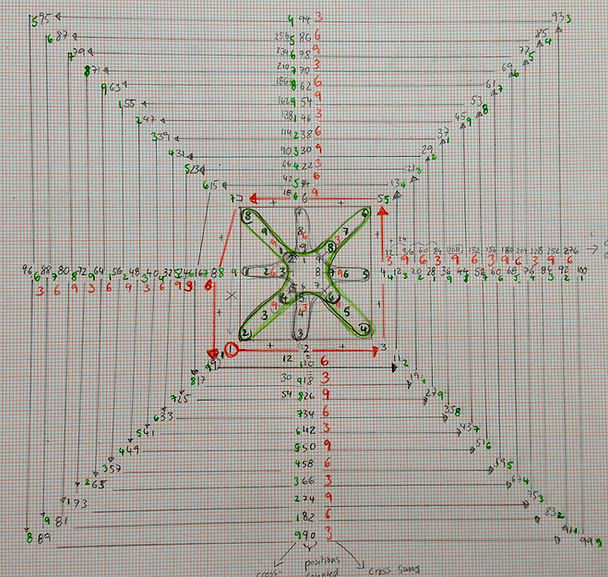Circumambulating the Square

In 'The Detail and the Whole' that which hangs stands - and vice versa. As the spectator circumambulates the form, every time s/he faces one of the four sides of what used to be the beam of wood / when s/he reaches 90, 180, 270 and 360 degrees of this circle (the centre of the 'sculpture' being the centre of the circle), the shape appears as the upside-down reversal of the previous position, creating a wave-like motion. Seen from what used to be the corner of the beam both aspects merge.

The detail / the contour - where the three straight line segments meet with the curved line sections- is identical with the Aleph (saddle) shape, and at the same time replicates the interlocking of the two half-circles at the centre of this 'sculpture'.Significance Of Number 9

Each 'sculpture' is based on a square. As the spectator circumambulates the vertical form s/he is offered a distinct number of views at each corner and surface of the wooden beam. When counting these distinct different aspects of each work starting from the corner of the beam a repeating sequence develops:Numbers written in pencil count the distinct views from corners and faces continuously spiraling outwards around the square: Starting at one corner with position 1, followed by the frontal view of a surface (2), followed by a corner view (3), and so on. When adding the positions of each side of the beam, the cross-sums of each of these sides result in a number sequence (marked in red in the diagram above), which repeats in each new round:

1+2+3         =  6

3+4+5        =  3

5+6+7        =  9

7+8+9        =  6

9+10+11      =  3

11+12+13     = 9

...

6+3+9 itself = 9

This sequence - 6 3 9 - only repeats if at the point of reaching the end of the first round, counting does not close the circle to return to position one, but instead extends out in a spiral-like manner into a second round - and continues with position no 9 instead

For the 639 sequence to repeat infinitely, counting must be continued in this spiral-like manner around the square and consequently away from or 'up' the length of the beam.

The cross-sums of each  of the counted positions (marked in green) reveal that the number sequence 1, 2, 3, 4, 5, 6, 7, 8, 9 repeats infinitely as the spiral continues to grow around the square (yet this sequence itself heads inwards - 1 being further on the periphery and 9 nearing the centre of the square):

1

2

3

4

5

6

7

8

9

10= 1+0 = 1

11 = 1+1 = 2

12= 1+2=  3

13= 1+3 = 4

14= 1+4= 5

15= 1+5 = 6

16=1+6 = 7

17=1+7  =8

18=1+8=  9

19=1+9=  1

...

Thus by circling the square 9 times one sequence is complete.

Only at one point/corner - at the original point of entry- the connection to the next round can be made. Thus one of the four corners of the square is different from the other three.

Once these sequences have been identified on the outside of the square, they can then be extended towards the inside of the square (diagram below).Inside the square:

Following the sequence of the counted positions around the outside of the square, consequently on the inside position 1 is followed by 2, position 2 followed by 3 and so on. When adding each of these pairs of the first outer and the first inner round together, the cross-sums (marked in pencil) are: 3, 5, 7, 9, 11, 13, 15 - and then not followed by 17, as the sequence would suggest, but 9 (!) instead.

The cross-sums for the two opposing sides of the diagram on

the left               (1+8) = 9

the right            (5+4) = 9

yet:

at the top            6+7 = 4

at the bottom     2+3 = 5

but added :         5+4 = 9

A trinity of 9's at the centre of the square.

And again the diagonal relationship as expressed in the values at opposing corners:

3+11  = 5

7+15 = 4

5+4  = 9

And again the cross-sums of the values along the sides is 9 :

11 + 13 + 15   = 9

3 + 5 + 7      = 9

3 + 9 + 15    = 9

7 + 9 + 11    = 9

Four sides of the square

4 x 9 = 36 = 9

9 comes after 8 and before 10.

10+8      = 9

8+9+10 = 9

Complete numerical symmetry inside the square.

The diagram below magnifies the inside of the square with focus on the repeating number sequence from 1 to 9 at each corner and each side of the square. It is magnified to contain 9 numbers of these sequences on the inside of the square.

At the centre there is now: 1, 2, 3, 4, 5, 7, but then not followed and concluded by 8 but 9 again instead.

But the cross-sum of this sequence  1+2+3+4+5+6+7+9  =  37  = 10 = 1.

Whereas if the last digit was 8 (and thus again not closing the circle but stepping outside to continue in a spiral-like movement, the cross-sum would again be 9:                 1+2+3+4+5+6+7+8 =  36 = 9

A circle has got 360 degrees. Continuous division by half result in cross-sums the result of which is again 9:

360  degrees =9

180  degrees  =9

90   degrees  =9

45    degrees  =9

22.5 degrees  =9

11.25 degrees =9

...

But how does 9 relate to π ?

Early calculations / approximations for pi:

Nilakantha series:

When taking the cross sums of three digits in this series the result is again the repeating 369 sequence:

2+3+4=9

4+5+6=6

6+7+8=3

8+9+10=9

10+11+12=6

12+13+14=3

...

Leibniz / Gregory Formula:

Leibniz' proof of this formula was found by the quadrature of the circle.

Again the cross-sums add up to 9:

1+3+5      = 9

7+9+11    =9

13+15+17 =9

...

## 9 links circle and square ... it seems to 'represent' growth and movement whilst ensuring complete symmetry in both, square and circle. Whereas approximations to pi -and from there patterns of 9 and 369- were found by division or the quadrature of the circle, for the square these patterns were found by circling the square in an outwards spiralling additive manner.

...